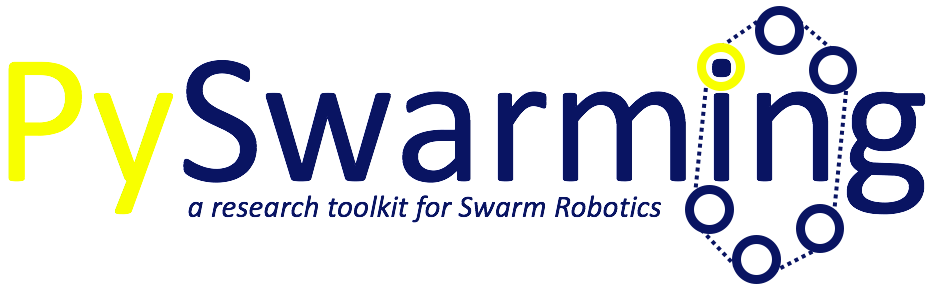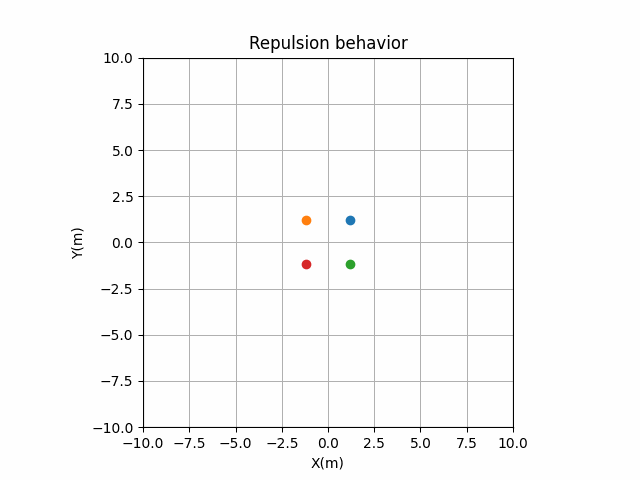# Welcome to pyswarming’s documentation!## Introduction

`pyswarming` is a research toolkit for Swarm Robotics.

The package is currently maintained by @mrsonandrade.

## Install

You can install `pyswarming` from PyPI using pip (Recommended):

```pip install pyswarming
```

## Dependencies

`pyswarming`’s dependencies are: `numpy` and `matplotlib`.

## Algorithms covered

This library includes the following algorithms to be used in swarm robotics:

• Inverse power: ajustable attraction and repulsion laws ;

• Spring: allows the robots to maintain a desired distance between them ;

• Force law: mimics the gravitational force ;

• Repulsive force: makes the individuals repulse each other ;

• Body force: introduces a body force that considers the radii of the robots ;

• Inter robot spacing: allows the robots to maintain a desired distance between them ;

• Dissipative: a dissipative force that reduces the “energy” of the robots ;

• Collision avoidance: the robot stays away from neighbors in the vicinity ;

• Attraction alignment: the robot becomes attracted and aligned ;

• Preferred direction: the robot has a preference to move toward a preset direction ;

• Lennard-Jones: allows the formation of lattices ;

• Virtual viscosity: a viscous force that reduces the “oscillation” of the robots ;

• Modified attraction alignment: the robot becomes attracted and aligned by considering a “social importance” factor ;

• Perimeter defense: the robots maximize the perimeter covered in an unknown environment ;

• Environment exploration: provides spatial coverage ;

• Aggregation: makes all the individuals aggregate collectively ;

• Alignment: the collective performs heading consensus ;

• Geofencing: attract the robots towards area A ;

• Repulsion: makes all the individuals repulse collectively ;

• Target: the robot goes to an specific target location ;

• Area coverage: using the Geofencing and Repulsion algorithms ;

• Collective navigation: using the Target and Repulsion algorithms ;

• Flocking: using the Aggregation, Repulsion and Alignment algorithms ;

References

## Examples using pyswarming.swarm

::

# importing the swarm creator import pyswarming.swarm as sw

### Repulsion

::
my_swarm = sw.Swarm(10, # number of robots

0.5, # linear speed of each robot 1.0, # sampling time [0.0, 0.0], # robots deployed randomly around x = 0.0, y = 0.0 (+- 5.0 meters) [[-50.0, 50.0], [-50.0, 50.0]], # plot limits x_lim, y_lim [‘repulsion’]) # list of behaviors

my_swarm.simulate()

::
my_swarm = sw.Swarm(10, # number of robots

0.5, # linear speed of each robot 1.0, # sampling time [0.0, 0.0], # robots deployed randomly around x = 0.0, y = 0.0 (+- 5.0 meters) [[-50.0, 50.0], [-50.0, 50.0]], # plot limits x_lim, y_lim [‘repulsion’]) # list of behaviors

my_swarm.behaviors_dict[‘r_out’][‘collective_navigation’][‘alpha’] = 2.0 # setting the strength of the repulsion my_swarm.behaviors_dict[‘r_out’][‘collective_navigation’][‘T’] = np.array([-40, -40, 0]) # setting the target my_swarm.simulate()

### Target + Aggregation

::
my_swarm = sw.Swarm(10, # number of robots

0.5, # linear speed of each robot 1.0, # sampling time [0.0, 0.0], # robots deployed randomly around x = 0.0, y = 0.0 (+- 5.0 meters) [[-50.0, 50.0], [-50.0, 50.0]], # plot limits x_lim, y_lim [‘target’,’aggregation’]) # list of behaviors

my_swarm.behaviors_dict[‘r_out’][‘target’][‘T’] = np.array([-40, -40, 0]) # setting the target my_swarm.simulate()

## Other Examples

Considering a swarm of robots, they can show different behaviors by using `pyswarming`. The following codes are simplified implementations, for detailed ones, see the Examples folder.:

```import pyswarming.behaviors as ps
import numpy as np
```

### Target

```# define the robot (x, y, z) position
r_i = np.asarray([0., 0., 0.])

# set the robot linear velocity
s_i = 1.0

# define a target (x, y, z) position
T = np.asarray([8., 8., 0.])

for t in range(15):

# print the robot (x, y, z) position
print(r_i)

# update the robot (x, y, z) position
r_i += s_i*ps.target(r_i, T)
```### Aggregation

```# define each robot (x, y, z) position
r = np.asarray([[8., 8., 0.],
[-8., 8., 0.],
[8., -8., 0.],
[-8., -8., 0.]])

# set the robot linear velocity
s_i = 1.0

for t in range(15):

# print the robot (x, y, z) positions
print(r)

# update the robot (x, y, z) positions
for r_ind in range(len(r)):
r_i = r[r_ind]
r_j = np.delete(r, np.array([r_ind]), axis=0)
r[r_ind] += s_i*ps.aggregation(r_i, r_j)
```### Repulsion

```# define each robot (x, y, z) position
r = np.asarray([[1., 1., 0.],
[-1., 1., 0.],
[1., -1., 0.],
[-1., -1., 0.]])

# set the robot linear velocity
s_i = 1.0

for t in range(15):

# print the robot (x, y, z) positions
print(r)

# update the robot (x, y, z) positions
for r_ind in range(len(r)):
r_i = r[r_ind]
r_j = np.delete(r, np.array([r_ind]), axis=0)
r[r_ind] += s_i*ps.repulsion(r_i, r_j, 3.0)
```### Aggregation + Repulsion

```# define each robot (x, y, z) position
r = np.asarray([[8., 8., 0.],
[-8., 8., 0.],
[8., -8., 0.],
[-8., -8., 0.]])

# set the robot linear velocity
s_i = 1.0

for t in range(15):

# print the robot (x, y, z) positions
print(r)

# update the robot (x, y, z) positions
for r_ind in range(len(r)):
r_i = r[r_ind]
r_j = np.delete(r, np.array([r_ind]), axis=0)
r[r_ind] += s_i*(ps.aggregation(r_i, r_j) + ps.repulsion(r_i, r_j, 5.0))
```